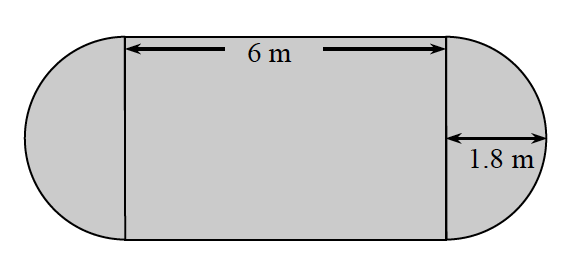### Home > ACC7 > Chapter 3 Unit 2 > Lesson CC3: 3.2.5 > Problem3-116

3-116.

Find the area and perimeter of the shape below. Show your work.Refer to the Math Notes box in Lesson 3.2.2 for review on circle area and circumference.

Since the radius of the circle is $1.8$ m, what is the diameter?

$3.6$ m
Now that you know the diameter, what is the width of the rectangle?

Now find the area of each separate shape.
Circle: $A=\pi·r^2$
Rectangle: (length)(width)

The total area of the shape is about $31.78\text{ m}^2$.
What is the perimeter?